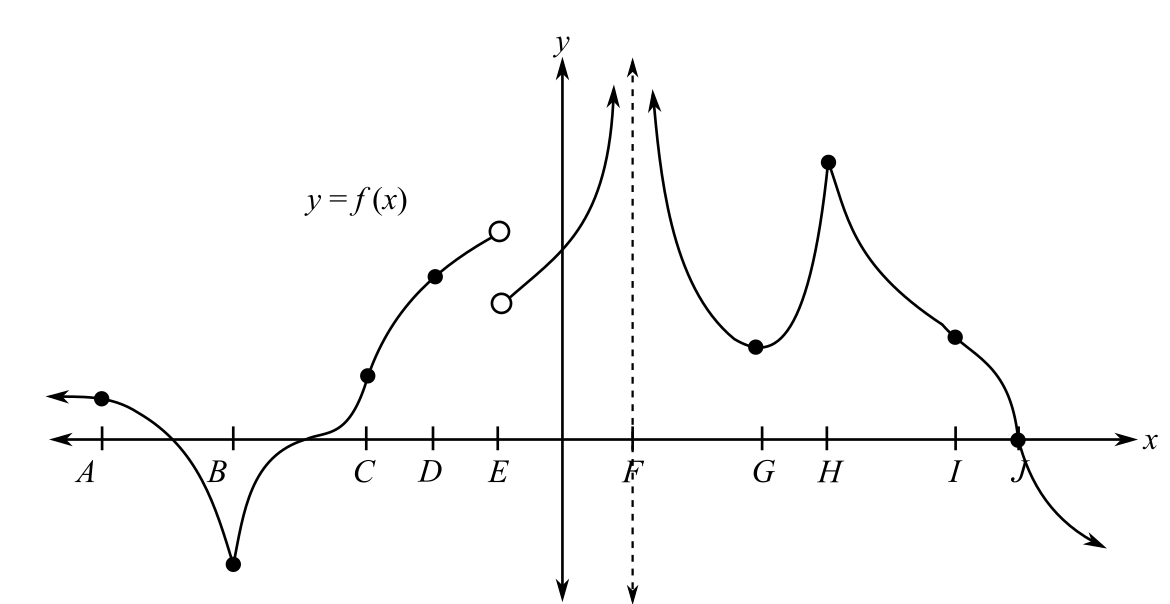Chapter 9.3, Problem 34E### Mathematical Applications for the ...

11th Edition
Ronald J. Harshbarger + 1 other
ISBN: 9781305108042

#### Solutions

Chapter
Section### Mathematical Applications for the ...

11th Edition
Ronald J. Harshbarger + 1 other
ISBN: 9781305108042
Textbook Problem

# Given the graph of y = f(x) in Figure 9.27, determine for which x-values F, G, H, I, or J the function is (a) continuous. (b) differentiable.(a)

To determine

The values of x out of F,G,H,I,J for which the function is continuous in the figure.Explanation

Given Information:

The graph is given as;

Explanation:

The graph is given as;

For a function to be continuous at a point, the left-hand limit should be equal to right hand limit and its value must be the value of the function at that point.

In case of graph, continuity of the left hand and right-hand limit is observed when the graph is continuous at the given point...

(b)

To determine

The values of x out of F,G,H,I,J for which the function is differentiable in the figure.### Still sussing out bartleby?

Check out a sample textbook solution.

See a sample solution

#### The Solution to Your Study Problems

Bartleby provides explanations to thousands of textbook problems written by our experts, many with advanced degrees!

Get Started

#### In Exercises 2340, find the indicated limit. 25. limx3x

Applied Calculus for the Managerial, Life, and Social Sciences: A Brief Approach

#### Evaluate the integral. 47. 1tan2xsec2xdx

Single Variable Calculus: Early Transcendentals

#### For y = x3 ln x, y = a) x2(1 + 3 ln x) b) x3 + 3x2 (ln x) c) 3x d) 4x2(ln x)

Study Guide for Stewart's Single Variable Calculus: Early Transcendentals, 8th

#### What is the primary factor that determines when it is time to change phases?

Research Methods for the Behavioral Sciences (MindTap Course List)